# Minimize Rastrigin's Function

### Rastrigin's Function

This example shows how to find the minimum of Rastrigin's function, a function that is often used to test the genetic algorithm. The example presents two approaches for minimizing: using the Optimize Live Editor task and working at the command line.

For two independent variables, Rastrigin's function is defined as

`$Ras\left(x\right)=20+{x}_{1}^{2}+{x}_{2}^{2}-10\left(\mathrm{cos}2\pi {x}_{1}+\mathrm{cos}2\pi {x}_{2}\right).$`

The `rastriginsfcn.m` file, which computes the values of Rastrigin's function, is available when you run this example. Create a surface plot of Rastrigin's function.

```fsurf(@(x,y)reshape(rastriginsfcn([x(:),y(:)]),size(x)),... "MeshDensity",100,... "ShowContours","on",... "LineStyle",":")```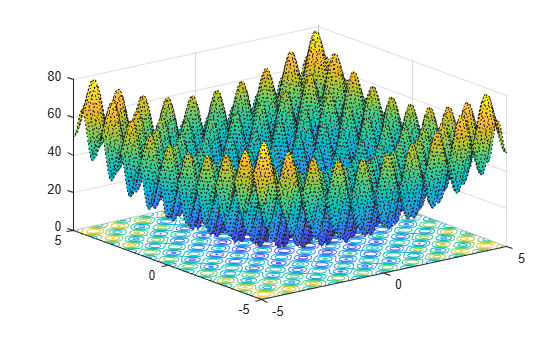As the plot shows, Rastrigin's function has many local minima—the “valleys” in the plot. However, the function has just one global minimum, which occurs at the point [0 0] in the x-y plane, where the value of the function is 0. At any local minimum other than [0 0], the value of Rastrigin's function is greater than 0. The farther the local minimum is from the origin, the larger the value of the function is at that point.

Rastrigin's function is often used to test the genetic algorithm, because its many local minima make it difficult for standard, gradient-based methods to find the global minimum.

### Minimize Using the Optimize Live Editor Task

This section explains how to find the minimum of Rastrigin's function using the genetic algorithm.

Note: Because the genetic algorithm uses random number generators, the algorithm returns different results each time you run it.

• Create a new live script by clicking the New Live Script button in the File section on the Home tab.• Insert an `Optimize` Live Editor task. Click the Insert tab and then, in the Code section, select Task > Optimize.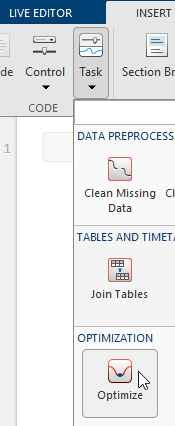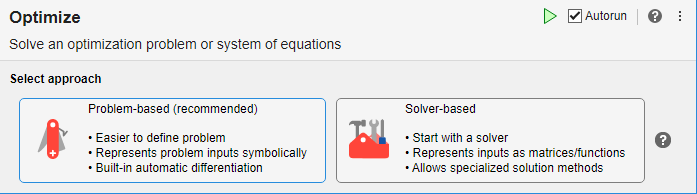• Click the Solver-based button.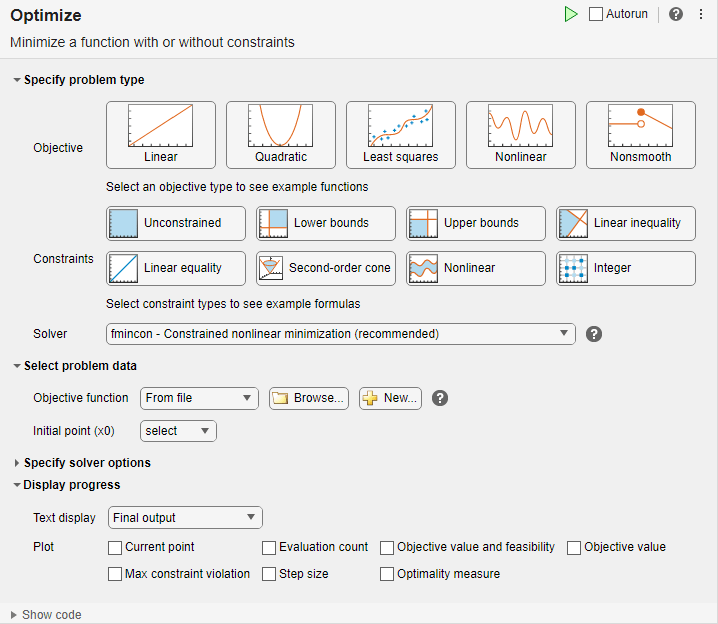• For use in entering problem data, insert a new section by clicking the Section Break button on the Insert tab. New sections appear above and below the task.

• In the new section above the task, enter the following code to define the number of variables and objective function.

```rng default % For reproducibility nvar = 2; fun = @rastriginsfcn;```
• To place these variables into the workspace, run the section by pressing Ctrl+Enter.

• In the Specify problem type section of the task, click the Objective > Nonlinear button.

• Select Solver > ga - Genetic algorithm.

• In the Select problem data section of the task, select Objective function > Function handle and then choose `fun`.

• Select Number of variables > nvar.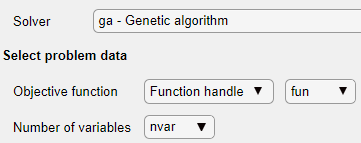• In the Display progress section of the task, select the Best fitness plot.

• To run the solver, click the options button at the top right of the task window, and select Run Section. The plot appears in a separate figure window and in the task output area. Note that your plot might be different from the one shown, because `ga` is a stochastic algorithm.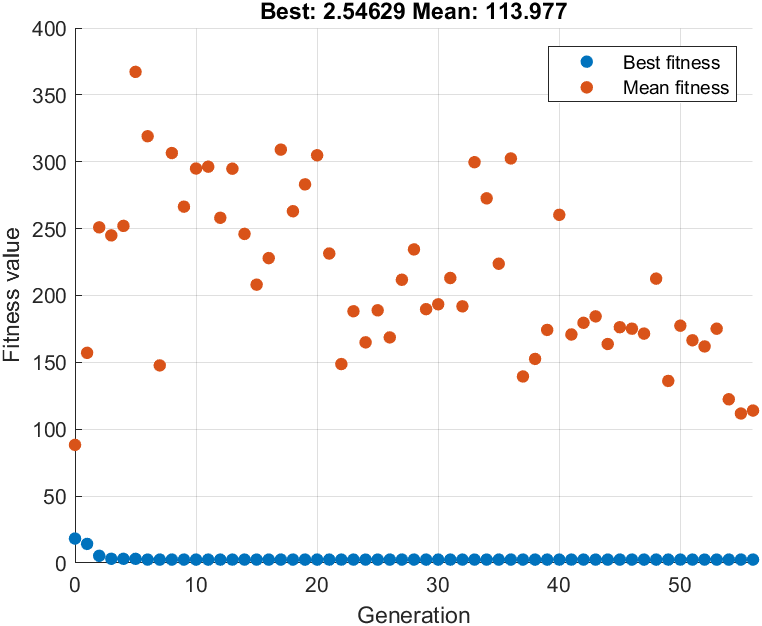• The points at the bottom of the plot denote the best fitness values, while the points above them denote the averages of the fitness values in each generation. The top of the plot displays the best and mean values, numerically, in the current generation.

• To see the solution and fitness function value, look at the top of the task.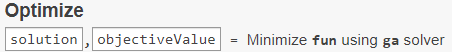• To view the values of these variables, enter the following code in the section below the task.

```disp(solution) disp(objectiveValue) ```

The value shown is not very close to the actual minimum value of Rastrigin's function, which is 0. The topics Set Initial Range, Vary Mutation and Crossover, and Set Maximum Number of Generations and Stall Generations describe ways to achieve a result that is closer to the actual minimum. Or, you can simply rerun the solver to try to obtain a better result.

The final state of the Live Editor task appears here.

```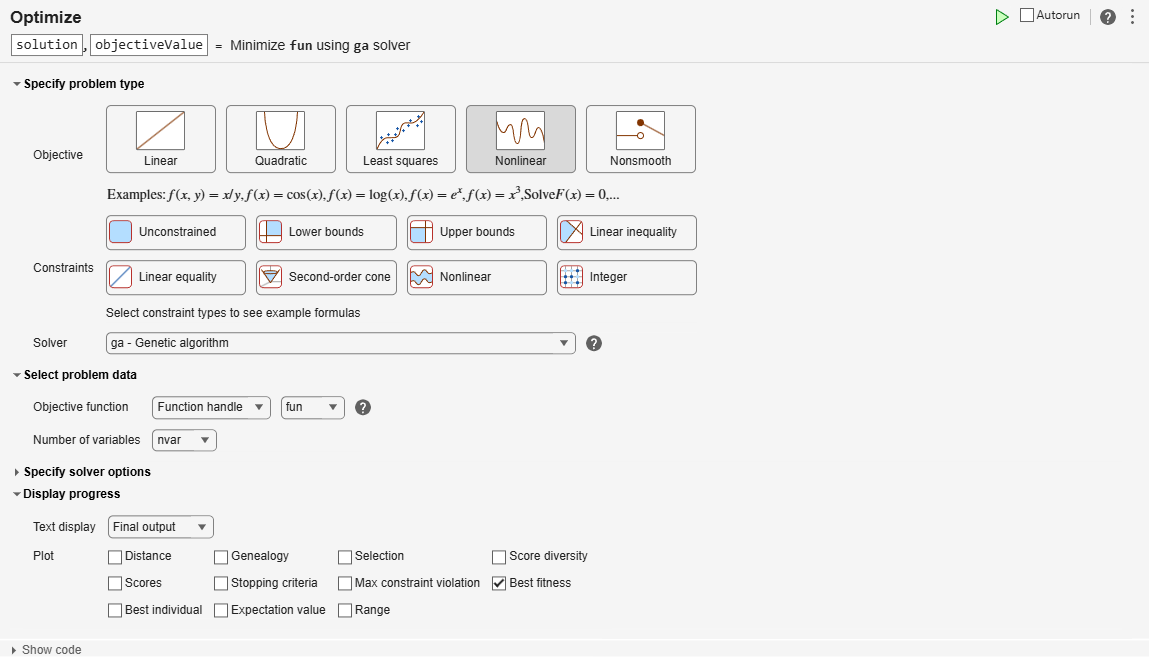```
```Optimization terminated: average change in the fitness value less than options.FunctionTolerance. ```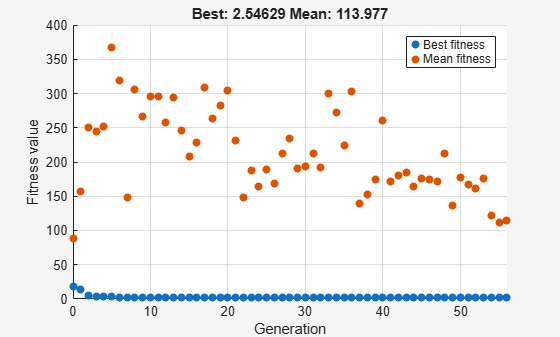`disp(solution)`
``` 0.9785 0.9443 ```
`disp(objectiveValue)`
``` 2.5463 ```

### Minimize at the Command Line

To find the minimum of Rastrigin's function at the command line, enter the following code.

```rng default % For reproducibility options = optimoptions('ga','PlotFcn','gaplotbestf'); [solution,objectiveValue] = ga(@rastriginsfcn,2,... [],[],[],[],[],[],[],options)```
```Optimization terminated: average change in the fitness value less than options.FunctionTolerance. ``````solution = 1×2 0.9785 0.9443 ```
```objectiveValue = 2.5463 ```

The points at the bottom of the plot denote the best fitness values, while the points above them denote the averages of the fitness values in each generation. The top of the plot displays the best and mean values, numerically, in the current generation.

Both the `Optimize` Live Editor task and the command line allow you to formulate and solve problems, and they give identical results. The command line is more streamlined, but provides less help for choosing a solver, setting up the problem, and choosing options such as plot functions. You can also start a problem using `Optimize`, and then generate code for command line use, as in Constrained Nonlinear Problem Using Optimize Live Editor Task or Solver.